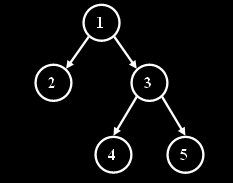### Problem Statement

The 0-th and 1-st Frabonacci trees are each single nodes. For all i > 1, the i-th Frabonacci tree is constructed as follows:

• Create a new node r. This will be the root node of the i-th Frabonacci tree.
• Construct the (i-1)-th and (i-2)-th Frabonacci trees.
• Attach the (i-2)-th Frabonacci tree as the left subtree of r.
• Attach the (i-1)-th Frabonacci tree as the right subtree of r.
The number of vertices in Frabonacci trees grows very quickly, for example there are about 4*10^10 vertices in the 50-th Frabonacci tree.

To traverse a Frabonacci tree in preorder, perform the following operations:

• Visit the root.
• Traverse the left subtree.
• Traverse the right subtree.
Let's traverse a Frabonacci tree and enumerate all vertices in the order of their visiting. There is the 3-rd Frabonacci tree with enumerated vertices on the picture.You are given three ints n, startIndex, finishIndex. Return the shortest route between the vertices startIndex and finishIndex in form of a String in the n-th Frabonacci tree. Each character of the result will be "L", "R" or "U", where "L" means a move to the left son, "R" means the move to the right son, and "U" means the move to the parent.

### Definition

 Class: FrabonacciTree Method: shortestPath Parameters: int, int, int Returns: String Method signature: String shortestPath(int n, int startIndex, int finishIndex) (be sure your method is public)

### Constraints

-n will be between 0 and 50, inclusive.
-startIndex will be between 1 and 1000000000, inclusive.
-finishIndex will be between 1 and 1000000000, inclusive.
-startIndex, finishIndex less or equal than the number of vertices in the n-th Frabonacci tree.

### Examples

0)

 `3` `2` `4`
`Returns: "URL"`
1)

 `3` `4` `2`
`Returns: "UUL"`
2)

 `3` `5` `4`
`Returns: "UL"`
3)

 `12` `10` `10`
`Returns: ""`

#### Problem url:

http://www.topcoder.com/stat?c=problem_statement&pm=8149

#### Problem stats url:

http://www.topcoder.com/tc?module=ProblemDetail&rd=10924&pm=8149

Mike Mirzayanov

#### Testers:

PabloGilberto , radeye , Olexiy , ivan_metelsky

Recursion